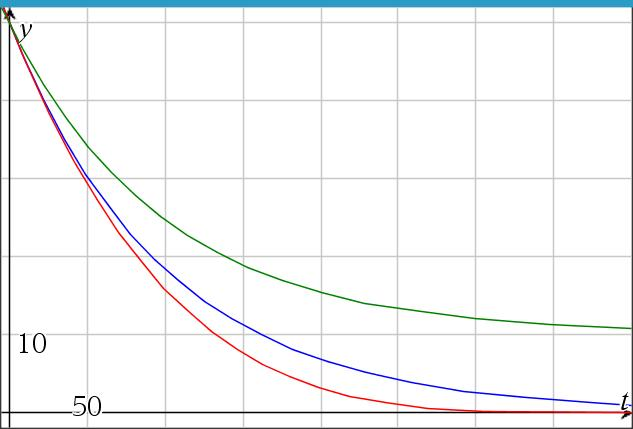# Brine mixture, case 3, inflow=outflow, brine in

Up a level : Differential Equations
Previous page : The limit of case 2
Next page : Brine mixture, case 4, inflow≠outflow, brine inCase 3: r1= r2=r, k=k1>0, V=V0

Now we have$\frac{{dy}}{{dt}} = kr - \frac{y}{V}r = r\left( {k - \frac{y}{V}} \right)$

This is separable. We get$\frac{{dy}}{{k - \frac{y}{V}}} = rdt$

Integrating both sides gives us$- V\ln \left( {k - \frac{y}{V}} \right) = rt + {C_1}$

or$\ln \left( {k - \frac{y}{V}} \right) = - \frac{r}{V}t + {C_2}$

And thus$k - \frac{y}{V} = {e^{ - \frac{r}{V}t + {C_2}}} = C{e^{ - \frac{r}{V}t}}$

This gives us$y = kV - C{e^{ - \frac{r}{V}t}}$

To find C:${y_0} = kV - C{e^{ - \frac{r}{V} \cdot 0}} = kV - C$

And thus$C = kV - {y_0}$

Finally:$y = kV - \left( {kV - {y_0}} \right){e^{ - \frac{r}{V}t}}$

We can see that case 1 is just a special case of case 3.

An example

Say we have V=V0=2000 l, r1 = r2 = r = 20 l/s, k=k1=10/2000 kg/l and y0 = 50 kg. This would give us the solution$y = 10 - \left( {10 - 50} \right){e^{ - \frac{t}{{100}}}} = 10 + 40{e^{ - \frac{t}{{100}}}}$

This can be seen as the green curve in the figure. We can see that we asymptotically get toward the concentration of the inflow.Up a level : Differential Equations
Previous page : The limit of case 2
Next page : Brine mixture, case 4, inflow≠outflow, brine inLast modified: Dec 28, 2020 @ 16:25